SLVSDA7E February   2017  – August 2019

PRODUCTION DATA.

1. Features
2. Applications
3. Description
1.     Device Images
4. Revision History
5. Device Comparison Table
6. Pin Configuration and Functions
7. Specifications
8. Detailed Description
1. 8.1 Overview
2. 8.2 Functional Block Diagram
3. 8.3 Feature Description
4. 8.4 Device Functional Modes
9. Application and Implementation
1. 9.1 Application Information
2. 9.2 Typical Application
1. 9.2.1 Design Requirements
2. 9.2.2 Detailed Design Procedure
3. 9.2.3 Setting the Current Limit
4. 9.2.4 Setting the Output Voltage
5. 9.2.5 TPS61178 Application Waveform
3. 9.3 System Examples
10. 10Power Supply Recommendations
11. 11Layout
12. 12Device and Documentation Support
1. 12.1 Device Support
2. 12.2 Documentation Support
5. 12.5 Community Resources
7. 12.7 Electrostatic Discharge Caution
8. 12.8 Glossary
13. 13Mechanical, Packaging, and Orderable Information

• RNW|13

#### 9.2.4.1 Selecting the Inductor

A boost converter normally requires two main passive components for storing the energy during the power conversion: an inductor and an output capacitor. The inductor affects the steady state efficiency ( including the ripple and efficiency ) as well as the transient behavior and loop stability, which makes the inductor to be the most critical component in application.

When selecting the inductor, as well as the inductance, the other parameters of importance are:

• The maximum current rating (RMS and peak current should be considered),
• The series resistance,
• Operating temperature

Choosing the inductor ripple current with the low ripple percentage of the average inductor current results in a larger inductance value, maximizes the converter’s potential output current and minimizes EMI. The larger ripple results in a smaller inductance value, and a physically smaller inductor, improves transient response but results in potentially higher EMI.

The rule of thumb to choose the inductor is that to make the inductor ripple current (ΔIL) is a certain percentage (Ripple % = 20 – 30 %) of the average current. The inductance can be calculated by Equation 5, Equation 6, and Equation 7:

Equation 5.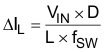Equation 6.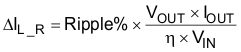Equation 7.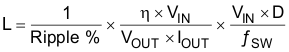where

• ΔIL is the peak-peak inductor current ripple
• VIN is the input voltage
• D is the duty cycle
• L is the inductor
• ƒSW is the switching frequency
• Ripple % is the ripple ration versus the DC current
• VOUT is the output voltage
• IOUT is the output current
• η is the efficiency

The current flowing through the inductor is the inductor ripple current plus the average input current. During power-up, load faults, or transient load conditions, the inductor current can increase above the peak inductor current calculated.

The TPS61178x has built-in slope compensation to avoid sub-harmonic oscillation associated with the current mode control. If the inductor value is too low and makes the inductor peak-to-peak ripple higher than 4 A, the slope compensation may not be adequate, and the loop can be unstable. Therefore, it is recommended to make the peak-to-peak current ripple below 4 A when selecting the inductor.

Inductor values can have ± 20% or even ± 30% tolerance with no current bias. When the inductor current approaches the saturation level, its inductance can decrease 20% to 35% from the value at 0-A bias current depending on how the inductor vendor defines saturation. When selecting an inductor, make sure its rated current, especially the saturation current, is larger than its peak current during the operation.

The inductor peak current varies as a function of the load, the switching frequency, the input and output voltages and it can be calculated by Equation 8 and Equation 9.

Equation 8.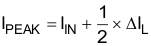where

• IPEAK is the peak current of the inductor
• IIN is the input average current
• ΔIL is the ripple current of the inductor

The input DC current is determined by the output voltage, the output current and efficiency can be calculated by :

Equation 9.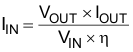where

• IIN is the input current of the inductor
• VOUT is the output voltage
• VIN is the input voltage
• η is the efficiency

While the inductor ripple current depends on the inductance, the frequency, the input voltage and duty cycle calculated by Equation 5, replace Equation 5, Equation 9 into Equation 8 and get the inductor peak current:

Equation 10.where

• IPEAK is the peak current of the inductor
• IOUT is the output current
• D is the duty cycle
• η is the efficiency
• VIN is the input voltage
• L is the inductor
• ƒSW is the switching frequency

The heat rating current (RMS) is as below:

Equation 11.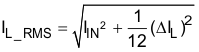where

• IL_RMS is the RMS current of the inductor
• IIN is the input current of the inductor
• ΔIL is the ripple current of the inductor

It is important that the peak current does not exceed the inductor saturation current and the RMS current is not over the temperature related rating current of the inductors.

For a given physical inductor size, increasing inductance usually results in an inductor with lower saturation current. The total losses of the coil consists of the DC resistance ( DCR ) loss and the following frequency dependent loss:

• The losses in the core material (magnetic hysteresis loss, especially at high switching frequencies)
• Additional losses in the conductor from the skin effect (current displacement at high frequencies)
• Magnetic field losses of the neighboring windings (proximity effect)

For a certain inductor, the larger current ripple (smaller inductor) generates the higher DC and also the frequency-dependent loss. An inductor with lower DCR is basically recommended for higher efficiency. However, it is usually a tradeoff between the loss and foot print.

The following inductor series in Table 2 from the different suppliers are recommended. 74437368033 from Würth is used for this application case with balancing the size and power loss.

### Table 2. Recommended Inductors for TPS61178x(1)

PART NUMBER L (μH) DCR Typ (mΩ) Max SATURATION CURRENT / Heat Rating Current (A) SIZE (L × W × H mm) VENDOR(1)
744325180 1.8 3.5 18 5 x 10 x 4 Würth
74437368033 3.3 11.8 23 / 8 10 x 10 x 3.8 Würth
DFEH10040D-3R3M# 3.3 12 10 / 10 10.9 x 10 x 4 Murata / TOKO
PIMB104T-4R7MS 4.7 20.0 15 / 8.5 10.9 x 10 x 3.8 Cyntec
74437368068 6.8 17.5 14 11 x 10 x 3.8 Würth
74437368100 10 27 12.5 11 x 10 x 3.8 Würth